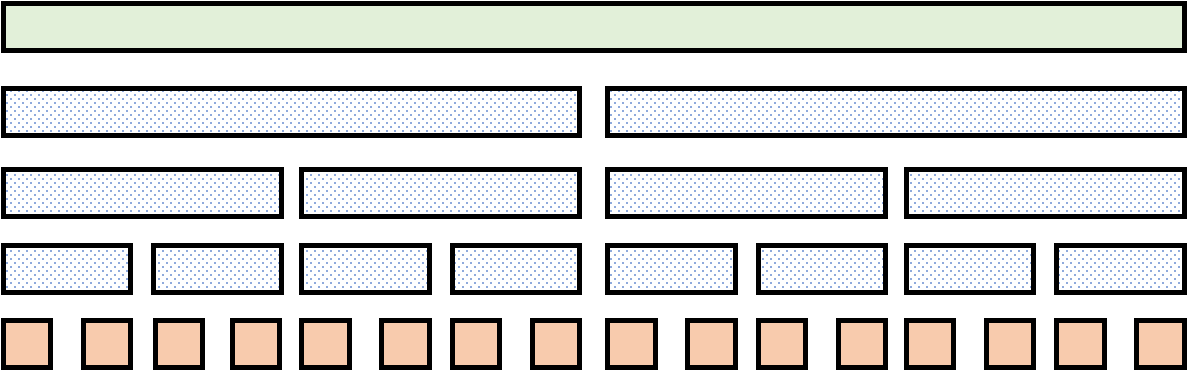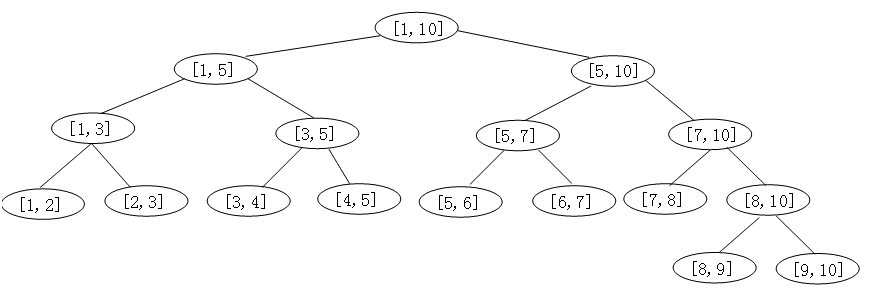# 基础线段树小记

## 初识线段树

### 线段树的一些基本性质

1. 普通线段树有 $O(n),\Theta(2n)$ 个节点，是一颗二叉树，如果除去深度最深的节点，是一颗满二叉树；
2. 线段树维护的信息应当是一个满足幺半群性质的信息
3. 线段树的每一个节点对应维护序列的一个区间。并且，一个节点 $[l,r]$ 的左儿子对应的节点是 $\big[l,\lfloor \frac {l+r}2 \rfloor\big]$，右儿子是 $\big(\lfloor \frac {l+r}2 \rfloor,r\big]$
4. 线段树的节点的信息应当是两个儿子信息的并，以保证计算不重不漏（因此线段树维护的信息应当是可以合并的）；
5. 线段树可以将一个区间分成 $O(\log n)$ 个极大的节点（区间）；
6. 由性质 1 可以得到线段树的深度是 $O(\log n)$ 的。

### 线段树的两种图像理解表示

1. 线段式（绿色的是根，橙色的是叶子结点，第 $i$ 个叶子结点对应的是 $A_i$2. 有根树式 1### 线段树的基本操作

#### 区间查询

/// @brief 示例：区间查询对应线段树节点
/// @param L 需要查询的范围左端点
/// @param R 需要查询的范围右端点
void RangeQuery(int L,int R,int l,int r,int p) {
//这一条保证了最多分成 O(log n) 个节点
if(L<=l&&r<=R) {printf("Node number: %d\n",p); return ;}

int mid=(l+r)>>1;
if(L<=mid) RangeQuery(L,R,l,mid,p<<1);
if(mid<R) RangeQuery(L,R,mid+1,r,p<<1|1);
}


#### 单点修改

/// @brief 示例：维护区间和
void PushUp(int p) {
nodes_[p].data=nodes_[p<<1].data+nodes_[p<<1|1].data;
}


/// @brief 示例：单点增加
/// @param X 元素在序列的位置（下标）
/// @param delta 元素增加的值
void NodeAdd(int X,int delta,int l,int r,int p) {
if(l==r) {nodes_[p].data+=delta; return ;}
int mid=(l+r)>>1;
PushUp(p);
}


#### 区间修改

• 性质：假设一个节点有懒标记，那么它的懒标记一定比存在懒标记的祖先的懒标记获得时间更早。

/// @brief 示例：区间增加的 push down
/// @note l,r 并不一定要有，只是这个示例需要。
void PushDown(int p,int l,int r) {
int mid=(l+r)>>1;
nodes_[p<<1].data+=tag*(mid-l+1);
nodes_[p<<1|1].data+=tag*(r-mid);
tag=0;
}


/**
* @brief 示例：区间加操作
*
* @param L 需要修改的范围左端点
* @param R 需要修改的范围右端点
* @param delta 增量
*/
void RangeAdd(int L,int R,int delta,int l,int r,int p) {
if(L<=l&&r<=R) {
nodes_[p].data+=(r-l+1)*delta;
return ;
}
PushDown(p,l,r); //注意 push down！
int mid=(l+r)>>1;
PushUp(p);
}


#### 建树

/// @brief 示例：区间加法的建树
/// @param init_array_ 初始的序列
void TreeInitialConstruct(int *init_array_,int l,int r,int p) {
if(l==r) {nodes_[p].data=init_array_[l]; return ;}
int mid=(l+r)>>1;
TreeInitialConstruct(init_array_,l,mid,p<<1);
TreeInitialConstruct(init_array_,mid+1,r,p<<1|1);
PushUp(p);
}


Code

## 基础权值线段树与动态开点线段树

struct node {
int left_son,right_son;
int cnt;
...
node() {left_son=right_son=cnt=0;}
};
node nodes_[kSIZE]; //其实我的习惯是写指针然后 new 一个数组。
int nodes_pointer_; //这个在记得初始化为 0。
int NewNode() {return ++nodes_pointer_;}


## 线段树上二分

/// @brief 查找当前区间第 k 大。
/// @note 如果是动态开点线段树，保持原样即可。如果是离散化后的普通权值线段树，那么
///       left_son 就是 p<<1，right_son 就是 p<<1|1。
int BinarySearch_kth_Element(int k,int l,int r,int p) {
if(l==r) return l;
int mid=(l+r)>>1,cnt_now=nodes_[nodes_[p].left_son].cnt;
if(k<=cnt_now)
return BinarySearch_kth_Element(k,l,mid,nodes_[p].left_son);
return BinarySearch_kth_Element(k-cnt_now,mid+1,r,nodes_[p].right_son);
}


#### 例题：P3369 【模板】普通平衡树

• 如果问题不强制在线，离散化后，没必要动态开点，时间复杂度是 $O(m\log n)$ 的，空间复杂度是 $O(n)$ 的。
• 如果问题强制在线，那么只能使用动态开点权值线段树，时间复杂度和空间复杂度都是 $O(m\log V)$这种复杂度比较高的，就会被卡，参见 P6136 【模板】普通平衡树（数据加强版）

## 拓展：线段树与线性变换

### 区间矩阵乘法解决元素内部的元素相互影响的问题

#### 例题：P7453 [THUSCH2017] 大魔法师

1. $A_i\leftarrow A_i+B_i$,
2. $B_i\leftarrow B_i+C_i$,
3. $C_i\leftarrow C_i+A_i$,
4. $A_i\leftarrow A_i+v$,
5. $B_i\leftarrow vB_i$,
6. $C_i\leftarrow v$.

1. $\begin{bmatrix} 1&1&0&0\\ 0&1&0&0\\ 0&0&1&0\\ 0&0&0&1 \end{bmatrix}$
2. $\begin{bmatrix} 1&0&0&v\\ 0&1&0&0\\ 0&0&1&0\\ 0&0&0&1 \end{bmatrix}$
3. $\begin{bmatrix} 1&0&0&0\\ 0&1&0&0\\ 0&0&0&v\\ 0&0&0&1 \end{bmatrix}$

（注意这个矩阵是按照列变换推的，要注意一下遍历顺序。）

Code 2（矩阵展开优化，但没优化多少）。

### 动态 DP (DDP) 结合线段树

DP 转移的本质是线性变换，也就是矩阵乘法。对于某些 DP，把 DP 初始状态写成一个向量，转移的过程写作一个矩阵，那么最终状态就是这个向量依次乘以各个矩阵。

#### 例题：CF1814E Chain Chips

1 转载自 https://hylwxqwq.github.io 洛谷 @black_trees
2 采用了 Doxygen 注释
3 作者发巅
4 作者早期码风